# KSEEB Solutions for Class 7 Maths Chapter 8 Comparing Quantities Ex 8.2

Students can Download Chapter 8 Comparing Quantities Ex 8.2, Question and Answers, Notes Pdf, KSEEB Solutions for Class 7 Maths, Karnataka State Board Solutions help you to revise complete Syllabus and score more marks in your examinations.

## Karnataka State Syllabus Class 7 Maths Chapter 8 Comparing Quantities Ex 8.2

Question 1.
Convert the given fractional numbers to percents.
a) $$\frac{1}{8}$$
Solution:
Percent means per hundred. So multi-plied by 100.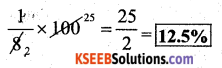b) $$\frac{5}{4}$$
Solution: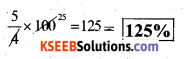c) $$\frac{3}{40}$$
Solution: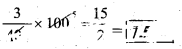d) $$\frac{2}{7}$$
Solution: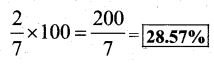Question 2.
Convert the given decimal fractions to per cents.
a) 0.65
Solution: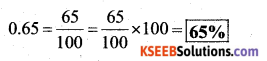b) 2.1
Solution: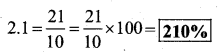c) 0.02
Solution: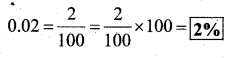d) 12.35
Solution: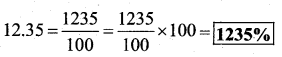Question 3.
Estimate what part of the figures is coloured and hence find the per cent which is coloured.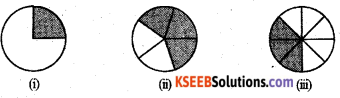Solution: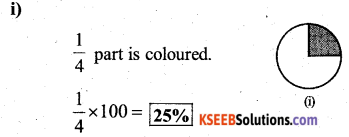∴ In the first figure 25% is coloured.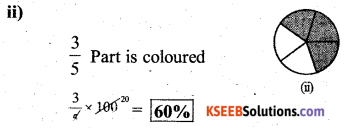∴ in the second figur e 50% Is coloured,.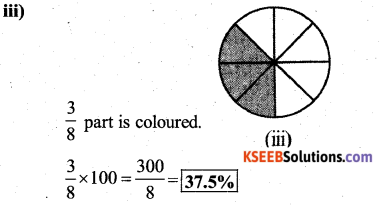∴ In the third figure 37.5 % is coloured.Question 4.
Find :
a) 15% of 250
Solution: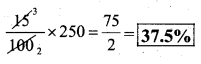b) 1% of 1 hour
Solution: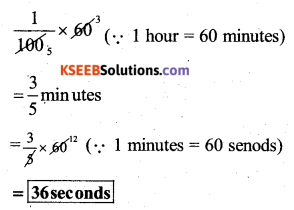c) 20% of ₹ 2500
Solution: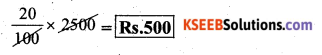d) 75% of ₹ 1 kg
Solution: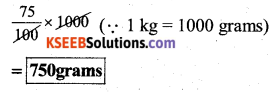Question 5.
Find the whole quantity if
a) 5% of it is 600.
Solution:
Let the whole quantity be ‘m’
∴ 5% of m = 600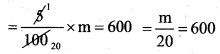∴ The whole quantity is 12,000.

b) 12% of its 1080.
Solution:
Let the whole quantity be ‘m’
∴ 12% of m = Rs. 1080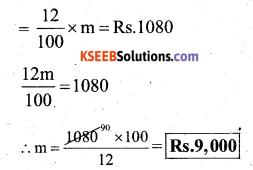∴ The whole amount be Rs. 9.000

c) 40 % of it is 500 km
Solution:
Let the whole quantity be ‘m’
∴ 40% of m = 500 km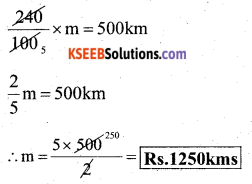∴ The whole amount is 1250 kmsd) 70% of it is 14 minutes
Let the whole quantity be ‘m’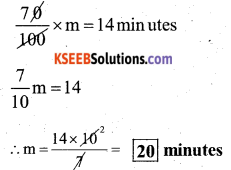∴ The whole amount is 20 minutes

e) 8% of it is 40 litres.
Let the whole quantity be ‘m’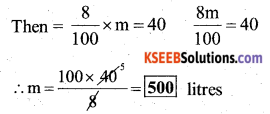∴ The whole amount be 500 litersQuestion 6.
Convert given per cents to decimal fractions and also to fractions in simplest forms:
Solution: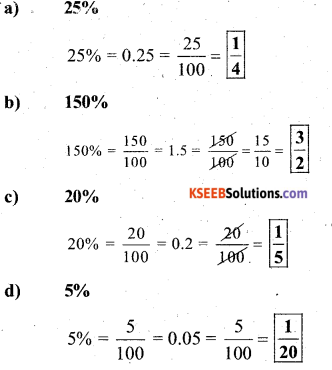Question 7.
In a city, 30% are females, 40% are males and the remaining are children. What percent are children?
Solution:
females = 30%
males = 40%
children = 100 – (30 + 40)
remaining are children = 100 – 70 = 30%
∴ Percentage of children = 30%Question 8.
Out of 15,000 votes in a constituency, 60% voted. Find the percentage of voters who did not vote. Can you now find how many actually did not vote?
Solution:
Total no. of voters in a constituency } = 15,000
Percentage of voted = 60%
∴ Percentage of voters who did not vote
= 100 – 60 = 40%
Actual number of voters who did not vote} = 40% of 15,000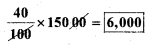6000 voters who did not vote.

Question 9.
Meeta saves ₹ 400 from her salary. If this is 10% of her salary. What is her salary?
Solution:
Let Meeta’s salary be ‘M’
10% of M is = Rs. 400
∴ M =?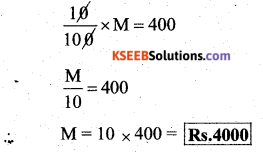∴ Her salary be Rs. 4,000/-Question 10.
A local cricket team played 20 matches in one season. It won 25% of them. How many matches did they win?
Let the total matches won by them be M 25% of 20 = M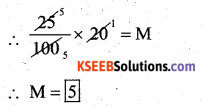∴ They win 5 matches.